# NCERT solutions for Class 11 Physics chapter 14 - Oscillations [Latest edition]

#### Chapters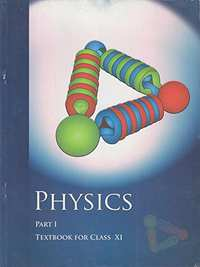## Solutions for Chapter 14: Oscillations

Below listed, you can find solutions for Chapter 14 of CBSE NCERT for Class 11 Physics.

Exercises
Exercises [Pages 357 - 361]

### NCERT solutions for Class 11 Physics Chapter 14 Oscillations Exercises [Pages 357 - 361]

Exercises | Q 1.1 | Page 357

Which of the following example represent periodic motion?

A swimmer completing one (return) trip from one bank of a river to the other and back.

Exercises | Q 1.2 | Page 357

Which of the following example represent periodic motion?

A freely suspended bar magnet displaced from its N-S direction and released.

Exercises | Q 1.3 | Page 357

Which of the following example represent periodic motion?

A hydrogen molecule rotating about its center of mass.

Exercises | Q 1.4 | Page 357

Which of the following example represent periodic motion?

An arrow released from a bow.

Exercises | Q 2.1 | Page 357

Which of the following example represent (nearly) simple harmonic motion and which represent periodic but not simple harmonic motion?

The rotation of the earth about its axis.

Exercises | Q 2.2 | Page 357

Which of the following example represent (nearly) simple harmonic motion and which represent periodic but not simple harmonic motion?

A motion of an oscillating mercury column in a U-tube.

Exercises | Q 2.3 | Page 357

Which of the following example represent (nearly) simple harmonic motion and which represent periodic but not simple harmonic motion?

The motion of a ball bearing inside a smooth curved bowl, when released from a point slightly above the lowermost point.

Exercises | Q 2.4 | Page 357

Which of the following example represent (nearly) simple harmonic motion and which represent periodic but not simple harmonic motion?

General vibrations of a polyatomic molecule about its equilibrium position.

Exercises | Q 3 | Page 357

Figure depicts four x-t plots for linear motion of a particle. Which of the plots represent periodic motion? What is the period of motion (in case of periodic motion)?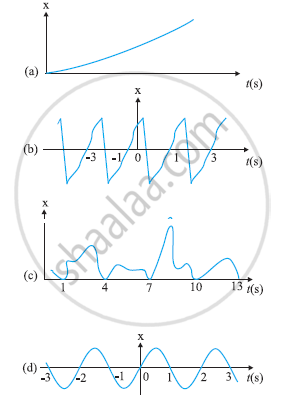Exercises | Q 4.1 | Page 358

Which of the following functions of time represent (a) simple harmonic, (b) periodic but not simple harmonic, and (c) non-periodic motion? Give period for each case of periodic motion (ω is any positive constant):

sin ωt – cos ωt

Exercises | Q 4.2 | Page 358

Which of the following functions of time represent (a) simple harmonic, (b) periodic but not simple harmonic, and (c) non-periodic motion? Give period for each case of periodic motion (ω is any positive constant):

sin3 ωt

Exercises | Q 4.3 | Page 358

Which of the following functions of time represent (a) simple harmonic, (b) periodic but not simple harmonic, and (c) non-periodic motion? Give period for each case of periodic motion (ω is any positive constant):

3 cos (π/4 – 2ω"t")

Exercises | Q 4.4 | Page 358

Which of the following functions of time represent (a) simple harmonic, (b) periodic but not simple harmonic, and (c) non-periodic motion? Give period for each case of periodic motion (ω is any positive constant):

cos ωt + cos 3ωt + cos 5ωt

Exercises | Q 4.5 | Page 358

Which of the following functions of time represent (a) simple harmonic, (b) periodic but not simple harmonic, and (c) non-periodic motion? Give period for each case of periodic motion (ω is any positive constant):

exp (–ω2t2)

Exercises | Q 4.6 | Page 358

Which of the following functions of time represent (a) simple harmonic, (b) periodic but not simple harmonic, and (c) non-periodic motion? Give period for each case of periodic motion (ω is any positive constant):

1 + ωt + ω2t2

Exercises | Q 5 | Page 358

A particle is in linear simple harmonic motion between two points, A and B, 10 cm apart. Take the direction from A to B as the positive direction and give the signs of velocity, acceleration and force on the particle when it is

(a) at the end A,

(b) at the end B,

(c) at the mid-point of AB going towards A,

(d) at 2 cm away from B going towards A,

(e) at 3 cm away from A going towards B, and

(f) at 4 cm away from B going towards A.

Exercises | Q 6 | Page 358

Which of the following relationships between the acceleration a and the displacement x of a particle involve simple harmonic motion?

(a) a = 0.7x

(b) a = –200x2

(c) a = –10x

(d) a = 100x3

Exercises | Q 7 | Page 359

The motion of a particle executing simple harmonic motion is described by the displacement function,

x (t) = cos (ω+ φ).

If the initial (= 0) position of the particle is 1 cm and its initial velocity is ω cm/s, what are its amplitude and initial phase angle? The angular frequency of the particle is π s–1. If instead of the cosine function, we choose the sine function to describe the SHM: x = B sin (ωt + α), what are the amplitude and initial phase of the particle with the above initial conditions.

Exercises | Q 8 | Page 359

A spring balance has a scale that reads from 0 to 50 kg. The length of the scale is 20 cm. A body suspended from this balance, when displaced and released, oscillates with a period of 0.6 s. What is the weight of the body?

Exercises | Q 9 | Page 359

A spring having with a spring constant 1200 N m–1 is mounted on a horizontal table as shown in Fig. A mass of 3 kg is attached to the free end of the spring. The mass is then pulled sideways to a distance of 2.0 cm and released.Determine (i) the frequency of oscillations, (ii) maximum acceleration of the mass, and (iii) the maximum speed of the mass.

Exercises | Q 10 | Page 359

let us take the position of mass when the spring is unstretched as x = 0, and the direction from left to right as the positive direction of the x-axis. Give as a function of time t for the oscillating mass if at the moment we start the stopwatch (= 0), the mass is

(a) at the mean position,

(b) at the maximum stretched position, and

(c) at the maximum compressed position.

In what way do these functions for SHM differ from each other, in frequency, in amplitude or the initial phase?

Exercises | Q 11 | Page 359

Figures correspond to two circular motions. The radius of the circle, the period of revolution, the initial position, and the sense of revolution (i.e. clockwise or anti-clockwise) are indicated on each figure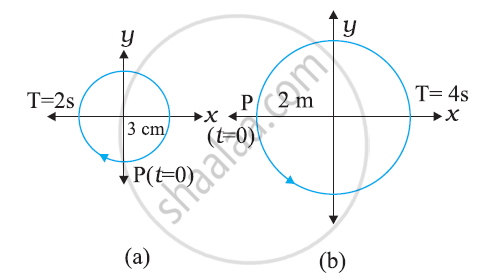Obtain the corresponding simple harmonic motions of the x-projection of the radius vector of the revolving particle P, in each case.

Exercises | Q 12.1 | Page 360

Plot the corresponding reference circle for the following simple harmonic motions. Indicate the initial (t = 0) position of the particle, the radius of the circle, and the angular speed of the rotating particle. For simplicity, the sense of rotation may be fixed to be anticlockwise in every case: (x is in cm and t is in s).

x = –2 sin (3t + π/3)

Exercises | Q 12.2 | Page 360

Plot the corresponding reference circle for the following simple harmonic motions. Indicate the initial (t = 0) position of the particle, the radius of the circle, and the angular speed of the rotating particle. For simplicity, the sense of rotation may be fixed to be anticlockwise in every case: (x is in cm and t is in s).

x = cos (π/6 - "t")

Exercises | Q 12.3 | Page 360

Plot the corresponding reference circle for the following simple harmonic motions. Indicate the initial (t = 0) position of the particle, the radius of the circle, and the angular speed of the rotating particle. For simplicity, the sense of rotation may be fixed to be anticlockwise in every case: (x is in cm and t is in s).

x = 3 sin (2πt + π/4)

Exercises | Q 12.4 | Page 360

Plot the corresponding reference circle for the following simple harmonic motions. Indicate the initial (t = 0) position of the particle, the radius of the circle, and the angular speed of the rotating particle. For simplicity, the sense of rotation may be fixed to be anticlockwise in every case: (x is in cm and t is in s).

x = 2 cos π t

Exercises | Q 13 | Page 360

Figure (a) shows a spring of force constant clamped rigidly at one end and a mass attached to its free end. A force F applied at the free end stretches the spring. Figure (b) shows the same spring with both ends free and attached to a mass mat either end. Each end of the spring in Fig. (b) is stretched by the same force F.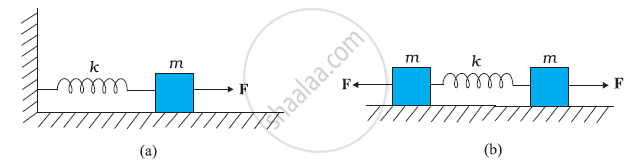(a) What is the maximum extension of the spring in the two cases?

(b) If the mass in Fig. (a) and the two masses in Fig. (b) are released, what is the period of oscillation in each case?

Exercises | Q 14 | Page 360

The piston in the cylinder head of a locomotive has a stroke (twice the amplitude) of 1.0 m. If the piston moves with simple harmonic motion with an angular frequency of 200 rad/min, what is its maximum speed?

Exercises | Q 15 | Page 360

The acceleration due to gravity on the surface of moon is 1.7 ms–2. What is the time period of a simple pendulum on the surface of moon if its time period on the surface of earth is 3.5 s? (on the surface of earth is 9.8 ms–2)

Exercises | Q 16.1 | Page 360

A time period of a particle in SHM depends on the force constant and mass of the particle: T = 2pi sqrt(m/k) A simple pendulum executes SHM approximately. Why then is the time

Exercises | Q 16.2 | Page 360

The motion of a simple pendulum is approximately simple harmonic for small angle oscillations. For larger angles of oscillation, a more involved analysis shows that is greater than 2pisqrt(1/g)  Think of a qualitative argument to appreciate this result.

Exercises | Q 16.3 | Page 360

A man with a wristwatch on his hand falls from the top of a tower. Does the watch give correct time during the free fall?

Exercises | Q 16.4 | Page 361

What is the frequency of oscillation of a simple pendulum mounted in a cabin that is freely falling under gravity?

Exercises | Q 17 | Page 361

A simple pendulum of length and having a bob of mass is suspended in a car. The car is moving on a circular track of radius with a uniform speed v. If the pendulum makes small oscillations in a radial direction about its equilibrium position, what will be its time period?

Exercises | Q 18 | Page 361

The cylindrical piece of the cork of density of base area and height floats in a liquid of density rho_1. The cork is depressed slightly and then released. Show that the cork oscillates up and down simple harmonically with a period

T = 2pi sqrt((hrho)/(rho_1g)

where ρ is the density of cork. (Ignore damping due to viscosity of the liquid).

Exercises | Q 19 | Page 361

One end of a U-tube containing mercury is connected to a suction pump and the other end to the atmosphere. A small pressure difference is maintained between the two columns. Show that, when the suction pump is removed, the column of mercury in the U-tube executes simple harmonic motion.

Exercises | Q 20 | Page 361

An air chamber of volume V has a neck area of cross section a into which a ball of mass m just fits and can move up and down without any friction (Fig.14.33). Show that when the ball is pressed down a little and released, it executes SHM. Obtain an expression for the time period of oscillations assuming pressure-volume variations of air to be isothermal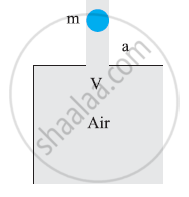Exercises | Q 21.1 | Page 361

You are riding in an automobile of mass 3000 kg. Assuming that you are examining the oscillation characteristics of its suspension system. The suspension sags 15 cm when the entire automobile is placed on it. Also, the amplitude of oscillation decreases by 50% during one complete oscillation. Estimate the values of the spring constant k.

Exercises | Q 21.2 | Page 361

You are riding in an automobile of mass 3000 kg. Assuming that you are examining the oscillation characteristics of its suspension system. The suspension sags 15 cm when the entire automobile is placed on it. Also, the amplitude of oscillation decreases by 50% during one complete oscillation. Estimate the values of the damping constant b for the spring and shock absorber system of one wheel, assuming that each wheel supports 750 kg.

Exercises | Q 22 | Page 361

Show that for a particle in linear SHM the average kinetic energy over a period of oscillation equals the average potential energy over the same period.

Exercises | Q 23 | Page 361

A circular disc of mass 10 kg is suspended by a wire attached to its centre. The wire is twisted by rotating the disc and released. The period of torsional oscillations is found to be 1.5 s. The radius of the disc is 15 cm. Determine the torsional spring constant of the wire. (Torsional spring constant α is defined by the relation = –α θ, where is the restoring couple and θ the angle of twist).

Exercises | Q 24.1 | Page 361

A body describes simple harmonic motion with an amplitude of 5 cm and a period of 0.2 s. Find the acceleration and velocity of the body when the displacement is 5 cm.

Exercises | Q 24.2 | Page 361

A body describes simple harmonic motion with an amplitude of 5 cm and a period of 0.2 s. Find the acceleration and velocity of the body when the displacement is 3 cm.

Exercises | Q 24.3 | Page 361

A body describes simple harmonic motion with an amplitude of 5 cm and a period of 0.2 s. Find the acceleration and velocity of the body when the displacement is 0 cm.

Exercises | Q 25 | Page 361

A mass attached to a spring is free to oscillate, with angular velocity ω, in a horizontal plane without friction or damping. It is pulled to a distance x0 and pushed towards the centre with a velocity v0 at time = 0. Determine the amplitude of the resulting oscillations in terms of the parameters ω, x0 and v0. [Hint: Start with the equation acos (ωt) and note that the initial velocity is negative.]

## Solutions for Chapter 14: Oscillations

Exercises## NCERT solutions for Class 11 Physics chapter 14 - Oscillations

Shaalaa.com has the CBSE Mathematics Class 11 Physics CBSE solutions in a manner that help students grasp basic concepts better and faster. The detailed, step-by-step solutions will help you understand the concepts better and clarify any confusion. NCERT solutions for Mathematics Class 11 Physics CBSE 14 (Oscillations) include all questions with answers and detailed explanations. This will clear students' doubts about questions and improve their application skills while preparing for board exams.

Further, we at Shaalaa.com provide such solutions so students can prepare for written exams. NCERT textbook solutions can be a core help for self-study and provide excellent self-help guidance for students.

Concepts covered in Class 11 Physics chapter 14 Oscillations are Force Law for Simple Harmonic Motion, Energy in Simple Harmonic Motion, Some Systems Executing Simple Harmonic Motion, Damped Simple Harmonic Motion, Forced Oscillations and Resonance, Displacement as a Function of Time, Periodic Functions, Oscillations - Frequency, Periodic and Oscillatory Motion, Simple Harmonic Motion (S.H.M.), Simple Harmonic Motion and Uniform Circular Motion, Velocity and Acceleration in Simple Harmonic Motion.

Using NCERT Class 11 Physics solutions Oscillations exercise by students is an easy way to prepare for the exams, as they involve solutions arranged chapter-wise and also page-wise. The questions involved in NCERT Solutions are essential questions that can be asked in the final exam. Maximum CBSE Class 11 Physics students prefer NCERT Textbook Solutions to score more in exams.

Get the free view of Chapter 14, Oscillations Class 11 Physics additional questions for Mathematics Class 11 Physics CBSE, and you can use Shaalaa.com to keep it handy for your exam preparation.

Share